# Dielectrics

Also found in: Dictionary, Thesaurus, Medical.
The following article is from The Great Soviet Encyclopedia (1979). It might be outdated or ideologically biased.

## Dielectrics

materials that are poor conductors of electric current.

The term “dielectric” was introduced by M. Faraday to describe materials through which electric fields can penetrate. In any substance placed in an electric field, its component electric charges (electrons, atomic nuclei) are subjected to the forces of the field. As a result, some of the charges move directionally, creating an electric current. The rest of the charges are redistributed in such a way that the centers of mass of the positive and negative charges shift in relation to one another. This process is known as polarization of the substance. The predominance of one of the two processes—electric conductivity or polarization—is used as a criterion in classifying materials as either insulators (dielectrics) or conductors (metals, electrolytes, plasma). The conductivity of dielectrics is negligible in comparison to that of metals. For dielectrics the specific resistance ρ is on the order of 108-1017 ohm-cm; for the metals, ρ is in the range of 10-6-10-4 ohm-cm. There is also an intermediate class of materials called semiconductors, the properties of which have elements of both the process of electric conductivity and the process of polarization.

Classical physics attempted to explain the quantitative difference in electric conductivity between solid dielectrics and metals by the fact that in metals there are always free electrons whereas in dielectrics all electrons are bound—that is, the electrons belong to individual atoms and an electric field does not free them, but merely displaces them slightly. However, this explanation is inaccurate. Modern quantum mechanical theory shows that a solid is something like a giant molecule in which every electron belongs to the whole crystal. This statement is as valid for dielectrics as it is for metals. Differences in the behavior of electrons in dielectrics and metals are caused by different types of electron distribution by energy level.

Electron energy in a solid cannot assume an arbitrary value. The energy ranges that an electron may possess (allowed bands) alternate with regions of energies (gaps) that an electron cannot accept (forbidden bands). On one hand, electrons tend to occupy the levels of lowest energy. On the other hand, there can be only one electron at any given state. As a consequence, electrons will fill energy levels starting from zero to some maximum value. In dielectrics the highest energy level filled by electrons coincides with the upper boundary of one of the allowed bands (see Figure 1). In metals, the highest energy level filled by electrons lies within the allowed band.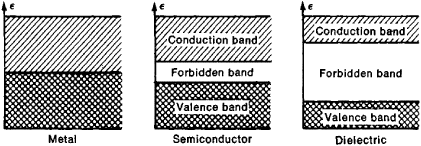Figure 1. Electron energy levels in solids, grouped in allowed (valence and conduction) bands and separated by forbidden bands

An electric field can establish a current (a directional motion of electrons) in a solid body only if some of the electrons can raise their energy in reponse to the field—that is, can move from lower energy levels to higher energy levels. Such a transition is possible in metals because empty energy levels are located adjacent to the filled levels. In dielectrics, however, the nearest empty levels are separated from the filled levels by the forbidden band. This forbidden band usually cannot be overcome by electrons under the influence of the not very strong conventional fields. Thus, in dielectrics, the effects of the electric field are limited to a redistribution of electron density, which results in polarization of the dielectric.

The distribution of electrons by energy levels is similar for semiconductors. A semiconductor differs from a dielectric only in that its forbidden band is narrower. Therefore, the properties of dielectrics and semiconductors are quite similar at low temperatures, but at elevated temperatures the electric conductivity of semiconductors increases and becomes significant. There is no clear borderline between dielectrics and semiconductors. Materials are classified as semiconductors if the width of the forbidden band Δ§ < 2–3 electron volts (eV), and as dielectrics if the width Δ§ > 2–3 eV.

The above discussion has been confined to solid dielectrics. However, dielectric materials can also be liquids or gases. Under normal conditions all gases consist basically of neutral atoms and molecules, and therefore do not conduct an electric current—that is, they are dielectrics. As the temperature of a gas is raised and the atoms and molecules become ionized, the gas is gradually converted to a plasma, a good conductor of electric current. The following discussion is limited to solid dielectrics.

Polarization of dielectrics. There are several mechanisms of polarization of a dielectric. These mechanisms depend on the nature of the chemical bond—that is, on the distribution of electron density in the dielectric. For example, in ionic crystals (for example, rock salt, NaCl) where the electrons are distributed such that individual ions can be isolated, polarization is the result of a shift of ions in relation to one another. This mechanism is called ionic polarization. Deformation of the electron shells of individual ions also occurs (electronic polarization). Stated differently, polarization in this case is the sum of ionic polarization and electronic polarization (see Figure 2, a). In crystals with a covalent bond (for example, in diamond) where electron densities are distributed uniformly among the atoms, polarization is predicated mainly on the displacement of the electrons that participate in chemical bonding (see Figure 2, b). In polar dielectrics (for example, solid hydrogen sulfide) groups of atoms—molecules or radicals—are electric dipoles; in the absence of an electrical field, these dipoles are oriented randomly, but under the influence of a field these dipoles are oriented in the direction of that field (see Figure 2, c). Such orientational polarization (or dipole polarization) is characteristic of polar liquids and gases. A similar mechanism of polarization is associated with a jump of ions from certain possible equilibrium sites in the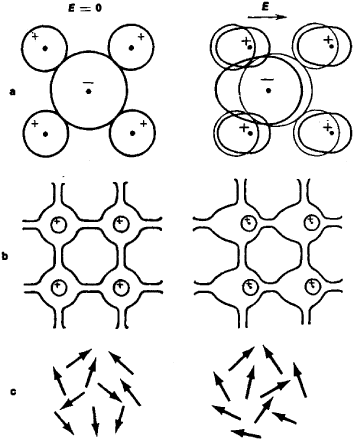Figure 2. Polarization of dielectrics in a field E. (a) Ionic and electronic polarization of ionic crystals, (b) electronic polarization of covalent crystals, (c) orientational polarization of polar dielectrics.

crystal lattice to others under the influence of an electric field. This mechanism is observed particularly often in materials with hydrogen bonding (for example, ice), where the hydrogen ions have several equilibrium positions.

Polarization of a dielectric is represented by the polarization vector ρ, which represents the dipole moment per unit volume of the dielectric. The dipole moment of an overall neutral system of charges is a vector equal to the product of the distance between the centers of mass of the positive and negative charges and the magnitude of one of these charges (either positive or negative). The direction of this vector is from the center of mass of the negative charges to the center of mass of the positive charges. The vector ρ depends on the electric field intensity E. Inasmuch as the force acting on a charge is proportional to the quantity E, it becomes obvious that for a weak field the magnitude of ρ is proportional to E. The proportionality constant K shown in the equation ρ = kE is called the dielectric susceptibility of the dielectric. It is often more convenient to substitute for the vector the vector of electric induction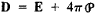The proportionality factor ∊ in the relationship D = ∊E is called the dielectric constant. It is clear that

∊ = 1 + 4πk

In a vacuum, K = 0 and ∊ = 1 (in the cgs esu system). The value of ∊ (or of K) is a basic characteristic of dielectrics.

In anisotropic dielectrics (for example, in noncubic crystals) the direction of the polarization vector is determined not only by the direction of the field ∊ but also by certain directions of the ambient medium, such as the axes of symmetry of a crystal. Therefore the vector ρ can be at various angles with vector E, depending on the orientation of ∊ in relation to the axes of symmetry. As a result, the vector D can be expressed through vector ∊ not only with one value ∊ but with several values (six in the general case), which form a dielectric permittivity tensor.

Dielectrics in an alternating field. If the electric field E changes with time, then the magnitude of polarization at a given moment t is not determined by the value of the field E at the same moment t. The polarization of a dielectric lags behind the change in electric field causing the polarization, since the displacement of charges cannot occur instantaneously (see Figure 3).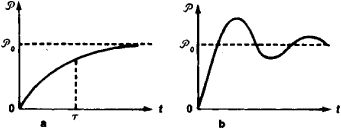Figure 3. Two types of polarization of a dielectric p as a function of time t; static electrical field ∊ is switched on at t = O

Any alternating field can be represented as a combination of fields changing according to a harmonic law (Fourier series, Fourier integral). Therefore, it is sufficient to study the behavior of dielectrics in a field E = E0 cos ωt, where ω is the frequency of the alternating field. Subjected to such a field, the values of D and ρ will oscillate harmonically at the same frequency ω. However, there will be a phase difference between the oscillations of D and E because of the lag of the polarization behind the field E.

The harmonic law can be stated in complex form as E = ρ. Then D = D0eiωt, wherein the amplitudes of oscillations of D and E are connected by the relationship D0 = ∊(ω). The dielectric constant ∊(ω) is, in this case the complex value ∊(ω) = ∊1+i#x128;2, characterized by the two values ∊1 and ∊2—both of which depend on the frequency o> of the alternating field. The absolute value ρ determines the amplitude of oscillation D, and the relation-ship (∊2/∊1) = tan δ determines the phase difference 8 between oscillations of D and E. The value Δ is called the dielectric loss angle, since the existence of the phase difference § leads to absorption of the energy of the electric field by the dielectric; indeed, the work done by the field E within a unit volume of the dielectric can be expressed by the integral ρ. For one cycle of oscillation this integral vanishes, if ρ and E are oscillating either in phase (§ = 0) or out of phase (δ = π). In all other cases the value of the integral is not zero. The energy fraction lost per cycle of oscillation equals ∊2g. In a static electric field (where ω = 0),∊2 = 0 and ∊1 coincides with ∊.

In alternating electric fields of very high frequencies (for example, electromagnetic waves in the optical range) the properties of dielectrics are customarily characterized by the index of refraction n and the absorption coefficient k (rather than by ∊1 and ∊2). The index of refraction n is equal to the ratio of the rate of propagation of electromagnetic waves in a dielectric to that in a vacuum. The absorption coefficient k is a measure of electromagnetic wave attenuation in a dielectric. The values n, k, and ∊12 are related by the equation n + ik =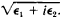Dispersion of the dielectric constant. The dependence of the dielectric constant on the frequency ω of an alternating field ∊(ω) = ∊1(ω)+i∊2(ω) is called the dispersion of the dielectric constant. The nature of the dispersion is determined by a process of establishing polarization as a function of time. If the process of establishing polarization is a relaxation process (see Figure 3, a), the dispersion will have the shape shown in Figure 4, a. If the oscillation cycle of the electric field is long in comparison to the relaxation time τ (that is, the frequency ω is low in comparison to 1/ω), polarization immediately follows the field. In this case the behavior of a dielectric in an alternating electric field is not essentially different from its behavior in a static field (that is, ∊,1 = ∊ and ∊2 = 0, as.shown in Figure 3, a). At frequencies ρ the dielectric does not have time for polarization—that is, the amplitude of ρ is very small in comparison to the magnitude of polarization of ρ in a static field. This means that ∊1 ≈ 1 and ∊2 ≈ 0. Thus, as the frequency increases, ∊1 changes from ∊ to 1. The sharpest change in ∊1 occurs exactly at frequencies ρ. At the same frequencies ∊2 passes through its maximum. This kind of dispersion of ∊(ω) is called relaxational dispersion. If the polarization undergoes oscillations during establishment such as that shown in Figure 3, b, the dispersion ∊(ω) will have the shape shown in Figure 4, b. This is called resonance dispersion.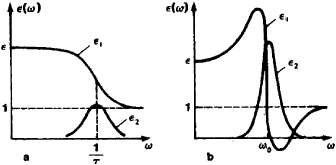Figure 4. (a) Relaxational dispersion of dielectric constant ∊ (ω), cor-responding Ƥ(t) in Figure 3, a; (b) resonance dispersion of dielectric constant ∊ (ω), corresponding to Figure 3, b

In reality the dispersion ∊(ω) is more complex than that represented in Figure 4. The function ∊(ω), typical for a wide variety of solid dielectrics, is shown in Figure 5. This figure shows several clearly distinguishable regions of dispersion at different frequency ranges. The existence of such distinctly separated regions points to the fact that the polarization of a dielectric is influenced by several different mechanisms. For example, in ionic crystals, polarization can be described as the sum of the ionic and electronic polarizations. For ionic oscillations, a typical cycle takes ~ 10-13 sec. Therefore the dispersion ∊(ω) owing to ionic polarization occurs at frequencies of -1013 hertz (Hz) (the infrared range), and the dispersion is usually of the resonance type. At higher frequencies there is not sufficient time for ion displacement and the entire contribution to the polarization is made by electrons. For electron oscillations, the characteristic period is determined by the width of the forbidden band for the dielectric in question. If the energy of a photon ħω (ħ being Planck’s constant) becomes greater than the width of the forbidden band, the photon can be absorbed, causing the transition of an electron through the forbidden band. Consequently, electromagnetic waves are strongly absorbed at these frequencies (ω ~ 1015 Hz—the ultraviolet range); that is, the value of ∊2 increases sharply. At lower frequencies (specifically, in the frequency range of visible light) pure homogeneous dielectrics are usually transparent (in contrast to metals).

In polar dielectrics an orientation of dipoles occurs under the influence of an electric field. The typical times required to establish polarization for this kind of orientational mechanism are relatively large: τ ~ 10-6-10-8 sec (the range of ultrahigh frequencies). In this case the dispersion is usually relaxational. Thus, a study of the function ∊(ω) makes it possible both to obtain information about the properties of a dielectric and distinguish the contributions to polarization made by the various polarization mechanisms.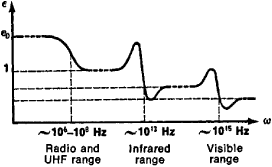Figure 5. ∊, of a solid dielectric as a function of frequency τ of field E

The dielectric constant of various materials. The static value of the dielectric constant ∊ depends significantly not only on the structure of the substance but also on external conditions, such as temperature, varying usually between 1 and 100–200 (for ferroelectric materials, to 104-105; see Table 1).

Table 1. Dielectric constant ∊ of some solid dielectrics
Dielectrics
Rock salt, NaCl...............6.3
Rutile, Ti02 (along optical axis)...............170
Diamond, C...............5.7
Quartz, SiO2...............4.3
Ice, H2O (at -5°C)...............73
Barium titanate, BaTi03 (at 20°C, perpendicular to optical axis)...............4,000

Such a spread of values for ∊ can be explained by the fact that in various substances the main contribution to ∊ at low frequencies is due to several different mechanisms of polarization. In ionic crystals the most important mechanism is that of ionic polarization. At high frequencies (ω ≤ 1014 Hz) the values of ∊(ω) for various ionic crystals approach 1, since only electronic polarization, the contribution of which is small, takes place at these frequencies. In covalent crystals, where the principal contribution to polarization is due to the redistribution of valence electrons, the static permittivity ∊ does not differ significantly from the high frequency permittivity ∊1(ω). Here the magnitude of ∊ depends on the “stiffness” of the covalent bond, which decreases as the forbidden band Δ becomes narrower. For example, for diamond (Δ = 5.5 eV), ∊ = 5.7. For silicon (Δ = 1.1 eV), ∊ = 12. A large part of ∊1 is due to orientational polarization. Therefore, in polar dielectrics, ∊ is comparatively large; for example, for water, ∊ = 81.

Methods of measuring the dielectric constant are different for different frequencies.

Polarization of dielectrics in the absence of an electrical field. So far, the discussion has been confined to dielectrics in which polarization arises under the influence of an external electric field. However, in a number of solid dielectrics polarization can be the result of other causes. In pyroelectrics polarization exists without the presence of an electric field. In these crystals the degree of asymmetry in the distribution of charges is such that the centers of mass of charges of opposite signs do not coincide—that is, the dielectric is polarized spontaneously. In piezoelectrics, polarization arises with deformation of the crystal; this phenomenon is associated with the structural peculiarities of its crystal lattice.

The ferroelectrics, a special variety of pyroelectrics, are of particular interest. One property exhibited by the ferroelectrics (but not by other pyroelectrics) is that spontaneous polarization varies significantly with changes in external conditions (temperature, electric field). The ferroelectrics characteristically show very high values for ∊, strong non-linear dependence of ρ on E, and domain structure; spontaneous polarization occurs only within a certain temperature range. In this regard, the dielectric properties of ferroelectrics are analogous to the magnetic properties of ferromagnetics.

Polarization in the absence of an electric field can also be observed in certain glasses and resins, called electrets. Electrets, which have been polarized at elevated temperatures and subsequently cooled, are capable of preserving their polarization for a considerable time without the presence of an electrical field.

The electric conductivity of dielectrics. The electric conductivity of dielectrics is low, although never equal to zero (see Table 2). Both electrons and ions can be current carriers in a dielectric. The electronic conductivity of a dielectric is determined by the same considerations as the electric conductivity of a semiconductor. However, under normal conditions the electronic conductivity of a dielectric is low in comparison to its ionic conductivity. Ionic conductivity may depend on the movement not only of the ions of the dielectric substance itself, but also of extrinsic impurity ions. The possibility of ion movement within a crystal is closely associated with the existence of crystal defects.. For example, if there are vacancies (unoccupied sites of the lattice) in the crystal, the presence of an electric field may cause an ion to jump into an adjacent vacant site, forming a new vacancy, which in turn can be occupied by another ion, and so on. The result is a migration of vacancies, which leads to a migration of the charge through the whole crystal. Ion migration can also occur as a result of ion jumps along the interstices. At elevated temperatures the ionic conductivity increases strongly. Surface conductivity may contribute markedly to the electric conductivity of a dielectric.

Table 2. Specific resistance ρ and electric strength Es of some solid dielectrics used as insulating materials
Dielectric materialρ, ohm.cmEs, V/cm
Quartz glass...............1016-10182-3 x 105
Polyethylene...............1015-10164 x 105
Mica...............1014-10161-2 x 105
Marble...............108-1092-3 x 105

Breakdown. In a dielectric, electric current is proportional to the electric field intensity E (Ohm’s law). However, if the field is sufficiently strong, electric current grows faster than indicated by Ohm’s law. An electric breakdown occurs when the field intensity reaches the critical value Es. This value Es is called the electric strength of a dielectric, or the dielectric strength (see Table 2). Upon breakdown, the uniformity of current distribution becomes unstable and almost all of the current begins to flow through a narrow channel. The current density j in this channel can attain very high values, leading to irreversible changes in the dielectric.

Figure 6 shows the dependence of current density j on the field intensity E, based on the assumption that the current density is uniform for a cross-sectional area of the sample. This relationship can be described by the equation j = (1/ρ)E, where the specific resistance ρ is not a constant value, as it would be in Ohm’s law, but a function of j. By differentiating the above equation, we obtain the expression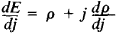from which it can be seen that if the value dρ/dj is negative, then as j increases, the value dE/dj may become negative (negative differential resistance). A state of negative differential resistance is unstable and leads to the formation of a current channel at E = Es.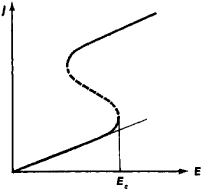Figure 6. Relationship between current density j and electric field intensity E in a dielectric; dotted line indicates unstable states

A distinction is made in solid dielectrics between thermal breakdown and electric breakdown. In a thermal breakdown the increase of j causes an increase in Joule heat, and thus an increase in the temperature of the dielectric; this leads to an increase in the number of current carriers n and a fall in the specific resistance ρ. In electric breakdown an increase in j also causes an increase in the number of current carriers n, and as n increases, ρ drops.

In practice the breakdown process is strongly affected by ever-present inhomogeneities. Such inhomogeneities promote breakdown, since E can experience localized increases at these sites. The formation of a current channel upon breakdown may cause several types of irreversible changes in the dielectric. For example, a hole through the dielectric may develop, or the dielectric may melt along the channel. Chemical reactions may also occur in the channel. For example, carbon may settle out in organic dielectrics, and metal may precipitate in ionic dielectrics (metallization of the channel).

The electric strength of liquid dielectrics depends strongly on the purity of the liquid. The presence of impurities and contaminants substantially lowers the Es, but for pure homogeneous liquid dielectrics the Es is nearly the same as the Es of solid dielectrics.

Breakdown in gases is associated with the mechanism of impact ionization. This breakdown manifests itself as an electrical discharge in gases.

Nonlinear properties of dielectrics. It has already been pointed out that polarization of a dielectric is proportional to electric field intensity. However, such a linear relationship holds true only for electric fields that are significantly weaker than the intracrystalline fields Ecr ~ 108 V/cm. Since normally Es <<Ecr, in the majority of dielectrics one does not find a nonlinear relationship ρ(E) in a static electric field. An exception to this rule occurs in ferroelectrics. These materials exhibit a strong nonlinear relationship ρ(E) within a certain temperature range (in the ferroelectric region and near phase transition points).

The electric strength of dielectrics increases at high frequencies. Therefore, the nonlinear properties of any dielectric show up in high frequency fields of high amplitude. In a laser beam electric fields can be generated with intensities of 108 V/cm. In such fields the nonlinear properties of dielectrics become very significant, which makes it possible to achieve such nonlinear effects as the frequency conversion and the self-focusing of light.

Dielectrics in science and technology. Dielectrics are used primarily as electrical insulators. In order to be used as insulators, dielectrics must have high specific resistance, high dielectric strength, and a low dielectric loss angle. Dielectrics with a high ∊ are used as capacitor materials. Filling a capacitor with a dielectric increases capacitance by a factor of e. Piezoelectrics are widely used to convert sound vibrations into electrical oscillations, and vice versa, in devices such as ultrasonic receivers and transmitters and sound pickups. Pyroelectrics are used in the detection and measurement of infrared radiation. Ferroelectrics are used to make nonlinear circuit elements in radio technology, such as amplifiers, frequency stabilizers, electric signal converters, and control circuits.

Dielectrics are also used in optics. Pure dielectrics are transparent in the visible range. Admixtures can be used to color a dielectric by making it opaque for a certain portion of the spectrum (as in a filter). Dielectric crystals are also used in quantum electronics (in quantum generators of light, such as lasers and ultrahigh frequency quantum mechanical amplifiers). Work is being conducted on the use of dielectrics in computer technology.

### REFERENCES

Feynman, R., R. Leighton, and M. Sands. Feinmanovskie lektsiipo fizike. [Issue 5.] Elektrichestvo i magnetizm. Moscow, 1966. (Translated from English.)
Kalashnikov, C. G. Elektrichestvo, 2nd ed. Moscow, 1964.
Fizicheskii entsiklopedicheskii slovar’, vol. 1. Moscow, 1960.
Skanavi, G. I. Fizika dielektrikov (Oblast’ slabykh polei). Moscow-Leningrad, 1949.
Skanavi, G. I. Fizika dielektrikov (Oblast’ sil’nykh polei). Moscow, 1958.
Frelikh, G., Teoriia dielektrikov. Moscow, 1960.
von Hippel, A. R. Dielektriki i volny. Moscow, 1960. (Translated from English.)
Zheludev, I. S. Fizika kristallicheskikh dielektrikov. Moscow, 1968.

A. P. LEVANIUK and D. G. SANNIKOV

References in periodicals archive ?
One of these laws is Lichtenecker's modeling law being used because of its general use  and its adequate interpretation of the composite materials dielectric behavior.
In July 2015, she filed a third-party action for negligence and respondeat superior against Dielectrics and Ramos.
At a high level, five variables are at work here, including trace width, copper weight, dielectric height, Dk and Df.
where [D.sub.2n], [D.sub.1n] are the normal components of the electric displacement vector: [D.sub.2n] = [[epsilon].sub.0][[epsilon].sub.2] [E.sub.2n], [D.sub.1n] = [[epsilon].sub.0][[epsilon].sub.1] [E.sub.1n] [epsilon].sub.0] = 8.85 [10.sup.-12] F/m is the electric constant; [[epsilon].sub.1], [[epsilon].sub.2] are the dielectric permeabilities of dielectrics, a is the surface density of electric charges; [E.sub.1n], [E.sub.2n] are the normal, [E.sub.1t], [E.sub.2t] are the tangential components of the electric field strength vector of the first and the second dielectrics, respectively.
Dielectric materials which can be used to control and/or store charge and electric energy play an important strategic role in modern electronic and power systems.
In recent decades, shale dielectric modeling was mainly focused on the effects of the electrical double layer associated with clay minerals (e.g., [1-7]).
Figure 2 shows [g.sub.m] versus [V.sub.G] characteristics of Si VDMOS with different gate dielectrics and different oxidation conditions before and after TID irradiation with [V.sub.G] = 10 V during irradiation.
Keywords: Dielectric Properties fillers loss peak Debye loss Peak Power law localization.
The smaller particles increase breakdown strength, and the larger particles increase the dielectric constant.
Table 1--Dielectric Constants Material Dielectric Constant, [epslion] Vacuum 1 (by definition) n-Hexane 1.9 PTFE 2.0 @ 60 Hz Polypropylene 2.2-2.3 @ 1 kHz Xylene 2.4 Epoxies ~3 PMMA 3.6 @ 50 Hz Alkyds ~4 n-Butyl acetate 5 Neoprene rubber 6.5-8.1 @ 1 kHz Talc ~ 8 MAK 15 n-Butanol 17.8 Ethanol 24 Methanol 33 Carbon black ~60 Dl water 80 R900 Ti[O.sub.2] ~100 The measurement of the dielectric constant requires specialized equipment.
The demand of composite dielectric materials derived from heterogeneous systems where matrix phase is insulating polymer and the dispersed phase is conductive additives are increasingly gaining importance because of their potential applications in aerospace, electrical, and electronic fields such as shielding enclosures, capacitors, sensors, microwave absorber, etc.
Keywords: Microwave; Irradiation; Organic synthesis; Enzymatic, Dielectric properties.

Site: Follow: Share:
Open / Close